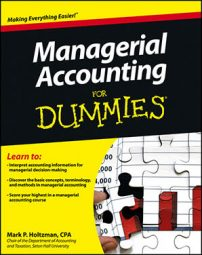##### Managerial Accounting For DummiesManagerial accountants understand that net present value (NPV) techniques use time value of money tools to estimate the current value of a series of future cash flows. Over time, the value of money changes. Given the choice between receiving \$1,000 today and receiving \$1,000 a year from now, most people would take the cash now because the value of money decreases with time. The later the cash flow, the less it’s worth.

Understanding and estimating how the value of money changes over time is the premise for evaluating the time value of money, an extremely important financial tool for making investment decisions.

For example, suppose you hit the lottery, winning \$1 million a year for the next 20 years. The state lottery board will publicize your winnings as a \$20 million prize, but that figure is misleading.

After all, time value of money principles say that the \$1 million received a year from now is somewhat less valuable than the \$1 million received today. The next installment, two years from now, would be worth even less than that, and so on. Therefore, simply multiplying \$1 million by 20 years disingenuously overstates the amount of the prize.

In fact, the net present value of a 20-year series of annual payments of \$1 million (assuming a 5-percent interest rate and that the first payment is received immediately) is equal to \$13,085,321. In other words, \$1 million a year for the next 20 years is really worth \$13,085,321 today.

Because net present value (NPV) techniques consider changes in the value of money, they offer an informative tool for managers making capital project decisions. After all, new investments, like a winning lottery ticket, should be expected to yield future cash inflows.

You have several options for computing the time value of money:

• Tables (found online and at the back of most managerial accounting textbooks)

• Formulas (which require familiarity with exponents)

• Microsoft Excel spreadsheets (which entail understanding how to use certain Excel formulas, such as NPV)

• Financial calculators (which have idiosyncratic commands explained in their instruction manuals)

Because the value of money decreases over time, use the variable PV (present value) to measure a cash flow today and the variable FV (future value) to estimate the value of a cash flow at some point in the future. Set the interest rate as variable i, expressed as a decimal (for example, 12 percent interest equals 0.12).